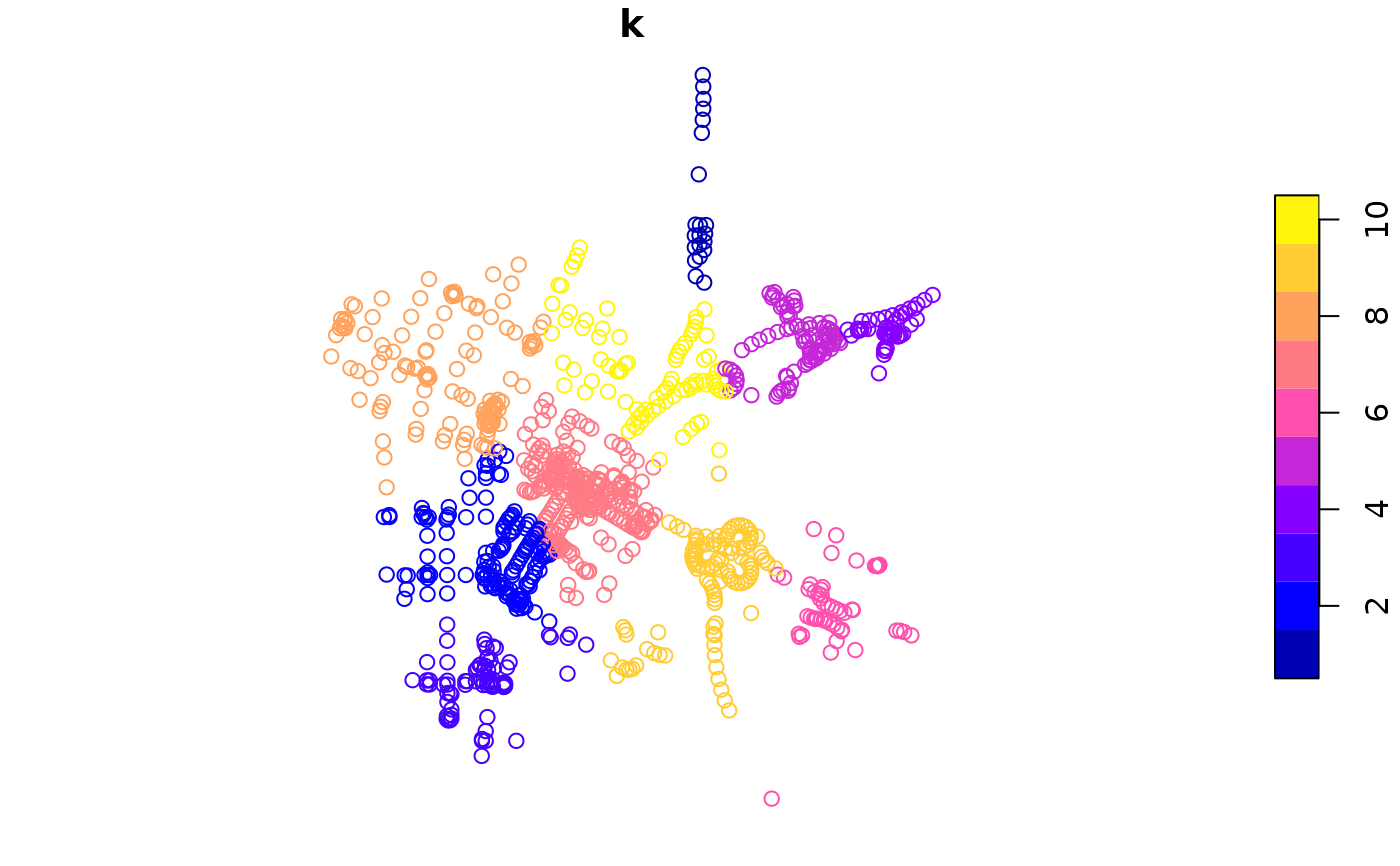This package is a simple R port (through V8) of a JavaScript library by Vladimir Agafonkin.

The concaveman function ports the concaveman library from mapbox. It computes the concave polygon for one set of points.

concaveman(points, concavity, length_threshold)

# S3 method for matrix
concaveman(points, concavity = 2, length_threshold = 0)

# S3 method for sf
concaveman(points, concavity = 2, length_threshold = 0)

## Arguments

points the points for which the concave hull must be computed. Can be represented as a matrix of coordinates or an sf object. a relative measure of concavity. 1 results in a relatively detailed shape, Infinity results in a convex hull. You can use values lower than 1, but they can produce pretty crazy shapes. when a segment length is under this threshold, it stops being considered for further detalization. Higher values result in simpler shapes.

## Value

an object of the same class as points: a matrix of coordinates or an sf object.

## Details

For details regarding the implementation, please see the original javascript library github page. This is just a thin wrapper, via V8.

## Examples

data(points)
polygons <- concaveman(points)
plot(points)plot(polygons, add = TRUE)